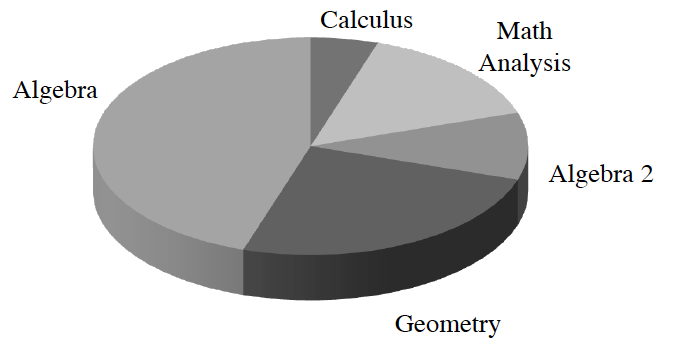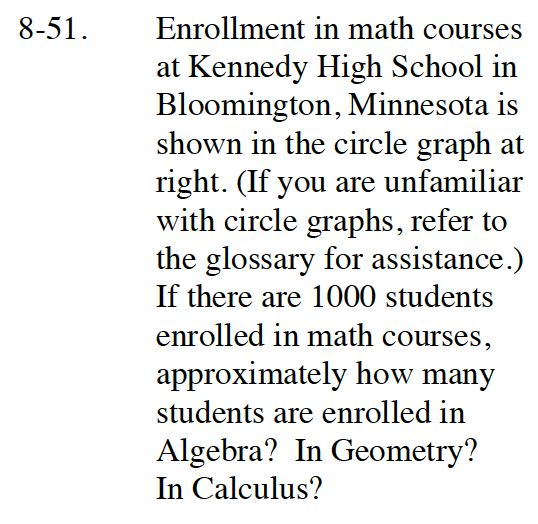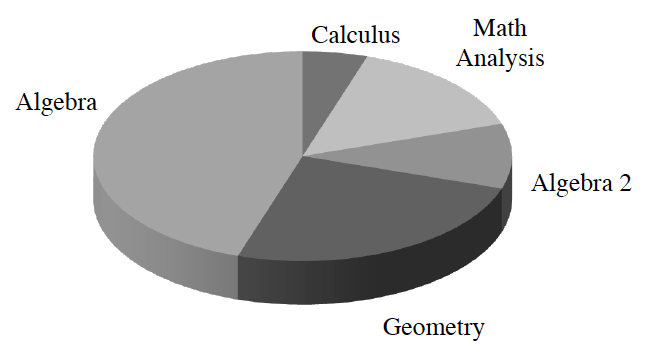Home > ACC7 > Chapter cc38 > Lesson cc38.2.1 > Problem8-51

8-51.Enrollment in math courses at Kennedy High School in Bloomington, Minnesota is shown in the circle graph at right. (If you are unfamiliar with circle graphs, refer to the glossary for assistance.) If there are 1000 students enrolled in math courses, approximately how many students are enrolled in Algebra? In Geometry? In Calculus? Homework Help ✎What approximate percentage of the circle graph is taken up by Algebra, Geometry, and Calculus?

Algebra: 450
Geometry: 250
Calculus: 50